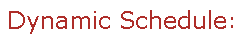Visit Minerva Class Schedule for course dates & times.

# MATH 318 Mathematical Logic (3 credits)

Offered by: Mathematics and Statistics (Faculty of Science)

### Overview

Mathematics & Statistics (Sci) : Propositional logic: truth-tables, formal proof systems, completeness and compactness theorems, Boolean algebras; first-order logic: formal proofs, Gödel's completeness theorem; axiomatic theories; set theory; Cantor's theorem, axiom of choice and Zorn's lemma, Peano arithmetic; Gödel's incompleteness theorem.

Terms: Fall 2022

Instructors: Sabok, Marcin (Fall)